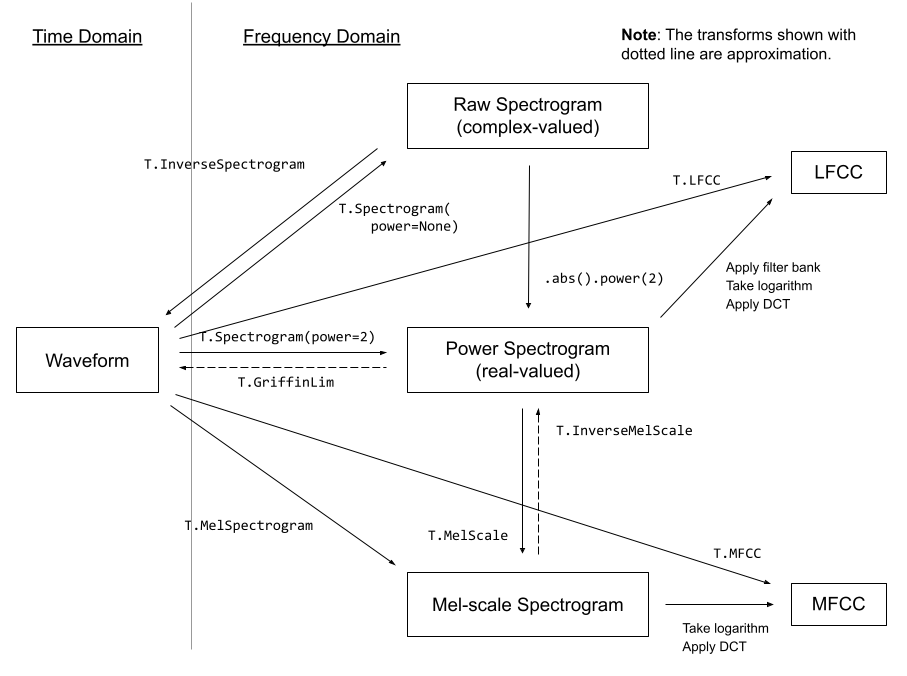Shortcuts

# torchaudio.transforms¶

torchaudio.transforms module contains common audio processings and feature extractions. The following diagram shows the relationship between some of the available transforms.Transforms are implemented using torch.nn.Module. Common ways to build a processing pipeline are to define custom Module class or chain Modules together using torch.nn.Sequential, then move it to a target device and data type.

# Define custom feature extraction pipeline.
#
# 1. Resample audio
# 2. Convert to power spectrogram
# 3. Apply augmentations
# 4. Convert to mel-scale
#
class MyPipeline(torch.nn.Module):
def __init__(
self,
input_freq=16000,
resample_freq=8000,
n_fft=1024,
n_mel=256,
stretch_factor=0.8,
):
super().__init__()
self.resample = Resample(orig_freq=input_freq, new_freq=resample_freq)

self.spec = Spectrogram(n_fft=n_fft, power=2)

self.spec_aug = torch.nn.Sequential(
TimeStretch(stretch_factor, fixed_rate=True),
)

self.mel_scale = MelScale(
n_mels=n_mel, sample_rate=resample_freq, n_stft=n_fft // 2 + 1)

def forward(self, waveform: torch.Tensor) -> torch.Tensor:
# Resample the input
resampled = self.resample(waveform)

# Convert to power spectrogram
spec = self.spec(resampled)

# Apply SpecAugment
spec = self.spec_aug(spec)

# Convert to mel-scale
mel = self.mel_scale(spec)

return mel

# Instantiate a pipeline
pipeline = MyPipeline()

# Move the computation graph to CUDA
pipeline.to(device=torch.device("cuda"), dtype=torch.float32)

# Perform the transform
features = pipeline(waveform)


Please check out tutorials that cover in-depth usage of trasforms.Audio Feature Extractions

Audio Feature Extractions

## Utility¶

 AmplitudeToDB Turn a tensor from the power/amplitude scale to the decibel scale. MuLawEncoding Encode signal based on mu-law companding. MuLawDecoding Decode mu-law encoded signal. Resample Resample a signal from one frequency to another. Fade Add a fade in and/or fade out to an waveform. Vol Adjust volume of waveform. Loudness Measure audio loudness according to the ITU-R BS.1770-4 recommendation. AddNoise Scales and adds noise to waveform per signal-to-noise ratio. Convolve Convolves inputs along their last dimension using the direct method. FFTConvolve Convolves inputs along their last dimension using FFT. Speed Adjusts waveform speed. SpeedPerturbation Applies the speed perturbation augmentation introduced in Audio augmentation for speech recognition . Deemphasis De-emphasizes a waveform along its last dimension. Preemphasis Pre-emphasizes a waveform along its last dimension.

## Feature Extractions¶

 Spectrogram Create a spectrogram from a audio signal. InverseSpectrogram Create an inverse spectrogram to recover an audio signal from a spectrogram. MelScale Turn a normal STFT into a mel frequency STFT with triangular filter banks. InverseMelScale Estimate a STFT in normal frequency domain from mel frequency domain. MelSpectrogram Create MelSpectrogram for a raw audio signal. GriffinLim Compute waveform from a linear scale magnitude spectrogram using the Griffin-Lim transformation. MFCC Create the Mel-frequency cepstrum coefficients from an audio signal. LFCC Create the linear-frequency cepstrum coefficients from an audio signal. ComputeDeltas Compute delta coefficients of a tensor, usually a spectrogram. PitchShift Shift the pitch of a waveform by n_steps steps. SlidingWindowCmn Apply sliding-window cepstral mean (and optionally variance) normalization per utterance. SpectralCentroid Compute the spectral centroid for each channel along the time axis. Vad Voice Activity Detector.

## Augmentations¶

The following transforms implement popular augmentation techniques known as SpecAugment .

 FrequencyMasking Apply masking to a spectrogram in the frequency domain. TimeMasking Apply masking to a spectrogram in the time domain. TimeStretch Stretch stft in time without modifying pitch for a given rate.

## Loss¶

 RNNTLoss Compute the RNN Transducer loss from Sequence Transduction with Recurrent Neural Networks .

## Multi-channel¶

 PSD Compute cross-channel power spectral density (PSD) matrix. MVDR Minimum Variance Distortionless Response (MVDR) module that performs MVDR beamforming with Time-Frequency masks. RTFMVDR Minimum Variance Distortionless Response (MVDR ) module based on the relative transfer function (RTF) and power spectral density (PSD) matrix of noise. SoudenMVDR Minimum Variance Distortionless Response (MVDR ) module based on the method proposed by Souden et, al. .

## Docs

Access comprehensive developer documentation for PyTorch

View Docs

## Tutorials

Get in-depth tutorials for beginners and advanced developers

View Tutorials